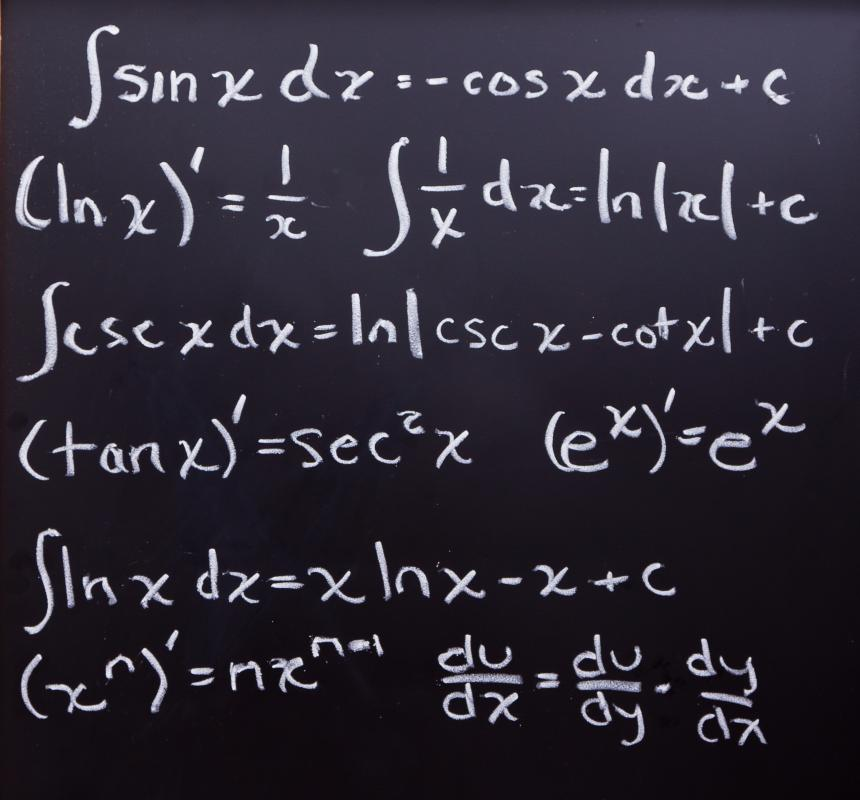# What are Common Derivatives?

Jeffrey L. Callicott
Jeffrey L. CallicottCommon derivatives are used in calculus.

In calculus, a derivative is a measure of the rate of change of a mathematical function. The term "common derivative" simply refers to a frequently seen type of derivative, or one that can be evaluated with relative ease. By contrast, complex derivatives are comparatively rare and can be difficult to calculate.

The majority of derivatives found in most mathematical applications are common derivatives. For instance, polynomials are functions comprised of everyday mathematical operators on a variable; some examples are 3x, x4, and 2x2 + 5x + 12. These are all polynomials because they are all functions that utilize the more frequently used mathematical operators on x. As a result, the derivatives of these and other, similar functions are considered common derivatives. Not only are the most basic rules of derivation used in their calculation, but more importantly, these functions are types more likely to be encountered.Derivatives, when used in calculus, measure the rate of change of a mathematical function.

When derivated, most widely utilized mathematical functions result in common derivatives. The derivatives for trigonometric functions are frequently seen and calculated relatively quickly. Other functions having derivatives that can be described as common are logarithms and functions that raise a number to a positive exponent.

There is a close relationship between common derivatives and common integrals. In much the same way that an integral is merely an antiderivative, common integrals are just common antiderivatives. Charts of common derivatives and integrals are usually present in most calculus textbooks and are available online.

Common derivatives find application as the foundation for most mathematical calculations involving rate of change. Velocity probably is the best known type of calculation for a rate of change. It is simply a derivative of position with respect to time; when an object is in motion, the rate of change of distance to another fixed or moving object can be calculated using a common derivative. A common derivative also can useful in determining the relative maximums or minimums of a function, which can help predict behaviors for any objects related to that function.

Although many people studying mathematics become adept at calculating common derivatives, real-world application tends to be more difficult. In such circumstances, it is sometimes helpful for one to determine what function could result in the described behavior. Another potentially useful way of attacking the problem is drawing a simple diagram of the portrayed situation. Either of these methods can betray the information necessary to arrive at a solution.

Derivatives are usually the first major new concept introduced to a student of calculus. Common derivatives are simple enough in concept that many formulas exist for their solutions. In spite of this, they remain one of the more obscure yet useful concepts in mathematics.

## You might also Like## Discuss this Article

Forgot password?
###### Register:
•Common derivatives are used in calculus.
•Derivatives, when used in calculus, measure the rate of change of a mathematical function.